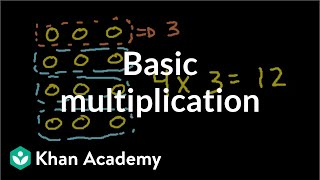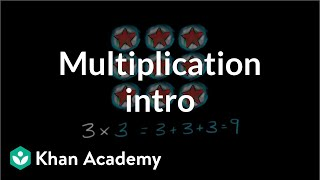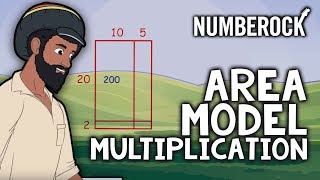HOME  |  TECHNOLOGY  |  HEALTH  |  FOOD  |  MORE

MULTIPLICATION

| Tutorials and interesting videos or articles about multiplication, numbers, multiplication games, memory, and decimals.VIDEO

Basic multiplication | Multiplication and division | Arithmetic | Khan Academy

Introduction to multiplicationVIDEO

Intro to multiplication | Multiplication and division | Arithmetic | Khan Academy

Use arrays and repeated addition to visualize multiplication.VIDEO

Area Model Multiplication ⋆ 2-Digit Multiplication by NUMBEROCK

Area Model Multiplication Song ⋆ 2-Digit by 2-Digit Multiplication ⋆ 4th- 5th GradeVIDEO

Box Method Multiplication

This short video walks through the Box Method algorithm for multiplication. This is a very helpful method for students who have difficulty with the traditional or standard algorithm, because it isolates the operations.